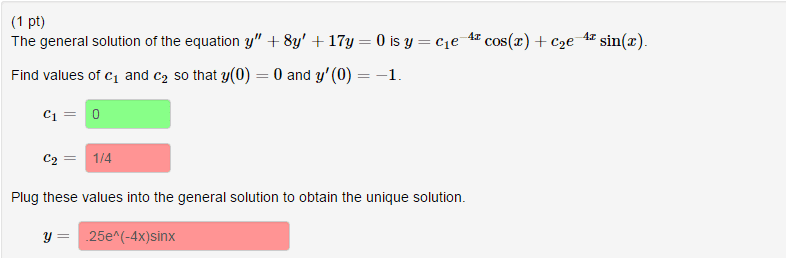# Find the constants for given IVP

## Homework StatementDifEqs

## The Attempt at a Solution

y ' = 4C1e-4xSinX - 4C2e-4xCosX

y'(0) = -1

-1 = 0 - 4C2

Therefore

C2 = 1/4

Not correct. What am I doing wrong?

vela
Staff Emeritus
Homework Helper
You didn't differentiate correctly. You have to use the product rule.

•Feodalherren
Sigh... obviously. Can't believe I just did that. Thanks!

Svein
What am I doing wrong?
1. $y=c_{1}e^{-4x}cos(x)+c_{2}e^{-4x}sin(x)$
2. $y'=c_{1}(-4e^{-4x}cos(x)-e^{-4x}sin(x))+c_{2}(-4e^{-4x}sin(x)+e^{-4x}cos(x))$
Now insert for y(0) and y'(0) and solve.

•Feodalherren
HallsofIvy
Personally, I would find it easier to write the solution as $y= e^{-4x}(C_1 cos(x)+ C_2 sin(x))$.
Then, by the product rule, $y'= -4e^{-4x}(C_1 cos(x)+ C_2 sin(x))+ e^{-4x}(-C_1 sin(x)+ C_2 cos(x))$.
•